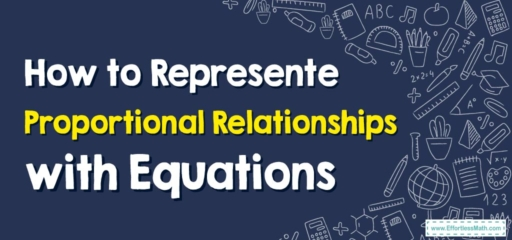# How to Represente Proportional Relationships with Equations

Proportional relationships are a fundamental concept in mathematics, with broad applications in areas ranging from simple ratio problems to more complex scientific or financial calculations. They represent a constant ratio between two variables.For example, if you’re driving at a constant speed, there’s a proportional relationship between the distance you’ve traveled and the time you’ve spent driving.
Representing proportional relationships with equations is a common way to visualize and analyze these relationships.

## A Step-by-step Guide to Representing Proportional Relationships with Equations

Here are the steps you can follow:

### Step 1: Understand the Concept of Proportional Relationships

A proportional relationship is one in which two quantities always have the same ratio. In other words, for any proportional relationship, a change in one quantity leads to a corresponding change in the other, by the same factor. For instance, if you have a proportional relationship where $$y$$ is twice as big as $$x$$, then when $$x$$ doubles, so does y. If $$x$$ is halved, so is $$y$$.

### Step 2: Identify a Proportional Relationship

This relationship can be identified in a variety of ways, such as through a graph, a table, or an equation.

• Table: A table shows a proportional relationship if the ratio of the quantities is the same for every pair of numbers.
• Graph: A graph of a proportional relationship is a straight line through the origin $$(0,0)$$.
• Equation: An equation represents a proportional relationship if it can be written in the form $$y=kx$$, where $$k$$ is the constant of proportionality.

### Step 4: Represent the Relationship with an Equation

Once you’ve found the constant of proportionality, you can write the equation that represents the relationship. If the constant of proportionality is $$3$$, for instance, the equation would be $$y=3x$$.

Here’s an example:

Let’s say you have a job where you earn $$15$$ for every hour you work.

The relationship between the hours you work and the money you earn is proportional because the more hours you work, the more money you make. The ratio of money earned to hours worked is always the same.

You can identify this relationship through an equation. Let’s let $$y$$ represent the money you earn and $$x$$ represent the hours you work. The ratio of the money you earn to the hours you work is $$\frac{y}{x}$$.

To find the constant of proportionality, you can take the amount of money you earn for an hour of work and divide it by the number of hours you worked. In this case, it’s $$\frac{15}{1}$$ hour $$=15$$ per hour.

Now you can represent this proportional relationship with an equation. The constant of proportionality $$(k)$$ is $$15$$, so the equation is $$y=15x$$. This means that for any number of hours, you work $$(x)$$, you can find out how much money you make $$(y)$$ by multiplying the hours by $$15$$.

That’s the basics of how to represent proportional relationships with equations!

### What people say about "How to Represente Proportional Relationships with Equations - Effortless Math: We Help Students Learn to LOVE Mathematics"?

No one replied yet.

X
30% OFF

Limited time only!

Save Over 30%

SAVE $5 It was$16.99 now it is \$11.99